Latest Banking jobs   »   Quantitative Aptitude Quiz For RBI Assistant/...

# Quantitative Aptitude Quiz For RBI Assistant/ ESIC UDC Mains 2022- 22nd April

Directions (1-5): In each of the following questions, three statements are given, you have to determine that which statement/statements is/are necessary to answer the question.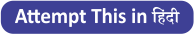Q1. A sum of money Rs 2550 is to be distributed among A, B and C. What will be the share of B?
A. A’s share is 1.5 times B’s share,
B. C’s share is half the share of A and B together.
C. The share of A is Rs 340 more than B.
(a) Either A and B together or A and C together are sufficient
(b) Only A and B together are sufficient
(c) Only A and C together are sufficient
(d) All statements are required
(e) Any 2 of the 3 statements are sufficient

Q2. 40 students sit in rows and columns. How many students are seated in each column?
A. The number of rows is 62.50% of the number of columns.
B. The number of rows is 5/8 of the number of columns.
C. The number of rows is less than the number of columns.
(a) Only A
(b) C and either A or B
(c) Only B
(d) Either A or B
(e) All the statements are required

Q3. A boat takes 2 hours to travel from point A to B in still water. To find out the speed upstream, which of the following information is/are required?
A. Distance between point A and B.
B. Time taken to travel downstream from B to A.
C. Speed of the stream of water.
(a) All are required
(b) Any one pair of A and B, B and C or C and A is sufficient.
(c) Only A and B
(d) Only A and C
(e) None of these

Q4. What is the price of a chair?
A. The price of three tables is equal to the price of seven chairs.
B. The difference between the price of a table and that of a chair is Rs 900.
C. The price of one table is 133⅓% more than the price of one chair.
(a) Only A and B together are sufficient
(b) Only A and C together are sufficient
(c) All together are necessary
(d) B and either A or C together are sufficient
(e) Even all A, B and C together are not sufficient

Q5. A bag contains balls of only three different colors i.e.; red, yellow and green. 3 balls are drawn randomly. What is the probability that the balls drawn are of three different colors?
A. The no. of yellow balls is two more than the no. of red balls.
B. The Sum of the no. of yellow and green balls is three times the no. of red balls.
C. The ratio of the no. of red balls to that of green balls is 3 : 4.
(a) A and either B or C
(b) Any two of them
(c) Only A and C together
(d) Question can’t be answered even after using all the information
(e) All statements are required

Directions (6-10): In the given questions, two quantities are given, one as ‘Quantity I’ and another as ‘Quantity II’. You have to determine relationship between two quantities and choose the appropriate option:

Q6. Train A cross a platform of length 520 meters in 22.8 sec and a man in 7.2 sec.
Quantity I -If train A cross train B running in same direction at 96 km/hr in 63 seconds then find the length of train B.
Quantity II – What is length of train C having speed of 90 km/hr and cross train A in 7.2 sec running in opposite direction.
(a) Quantity I > Quantity II
(b) Quantity I < Quantity II
(c) Quantity I ≥ Quantity II
(d) Quantity I ≤ Quantity II
(e) Quantity I = Quantity II or no relation

Q7. Ratio of age of Bhavya & Veer three years before was 7 : 8 and after six year it will be 10 : 11. Ankit is two years older than Bhavya, while Ayush is four years younger than that of Veer. The time when Bhavya and Veer completed their graduation, the ratio of their respective ages at that time was 20 : 23
Quantity I – Sum of age of Ankit & Ayush at the time when Bhavya & Veer respectively completed their graduation.
Quantity II – Average age of P, Q & R, two years hence will be 38 years and ratio of present age of Q & R be 16 : 9. P is 33 years old, ratio of age of R & T eight years hence will be 7 : 8. M is four years older than Q and ratio of present age of M & N is 13 : 14. Find average age of N & Q.
(a) Quantity I > Quantity II
(b) Quantity I < Quantity II
(c) Quantity I ≥ Quantity II
(d) Quantity I ≤ Quantity II
(e) Quantity I = Quantity II or no relation

Q8. Length of body diagonal of a cube is 60√3 cm, if cube is melted and formed some smaller cubes with side of 4 cm each or cube is melted and formed some cuboids of each having length of 8 cm, width of 5 cm & height of 2 cm.
Quantity I – Find the difference between increase in total surface area of all smaller cubes with respect to original cube & increase in total surface area of all cuboids formed with respect to original cube (in m²).
Quantity II – 3.16 m²
(a) Quantity I > Quantity II
(b) Quantity I < Quantity II
(c) Quantity I ≥ Quantity II
(d) Quantity I ≤ Quantity II
(e) Quantity I = Quantity II or no relation

Q9. A circle with center ‘o’ is circumscribed with an equilateral triangle ABC of side 24√3 cm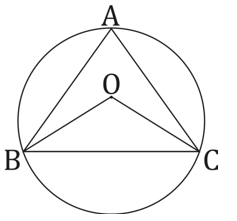Quantity I – Find the height of triangle.
Quantity II – Find the length of rectangle having perimeter 120 cm and breadth equal to radius of circle.
(a) Quantity I > Quantity II
(b) Quantity I < Quantity II
(c) Quantity I ≥ Quantity II
(d) Quantity I ≤ Quantity II
(e) Quantity I = Quantity II or no relation

Q10. Quantity I: A man has two solid balls. Ratio between radius of first ball and second ball is 4 : 3. If man cut the second ball from middle then difference between total surface area of first ball and total surface area of a part of second ball is 1424.5 cm². Find value of radius of bigger ball?
Quantity II: Height of a cylindrical vessel is equal to side of a square, having area is 256 cm². If volume of cylindrical vessel is 22176 cm³ then find the radius of cylindrical vessel.
(a) Quantity I > Quantity II
(b) Quantity I < Quantity II
(c) Quantity I ≥ Quantity II
(d) Quantity I ≤ Quantity II
(e) Quantity I = Quantity II or no relation

Solutions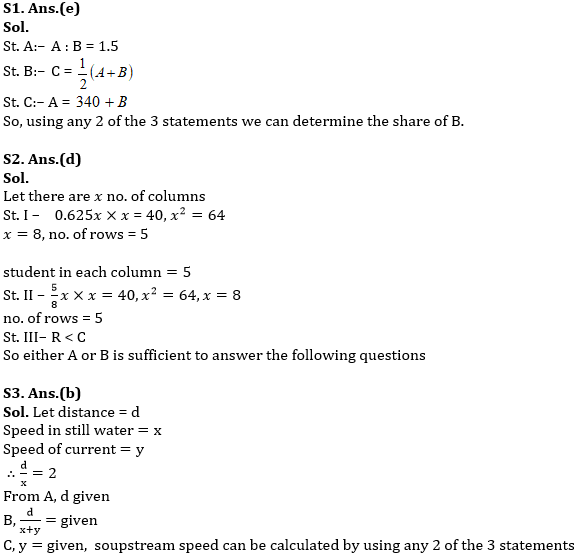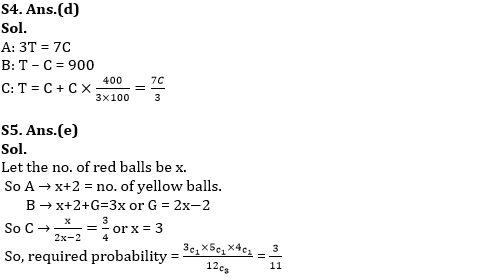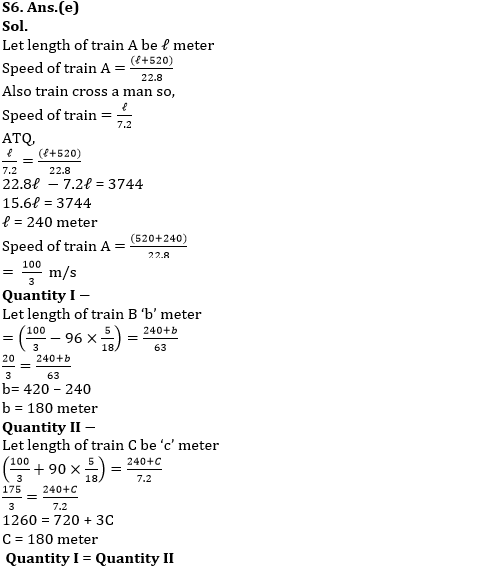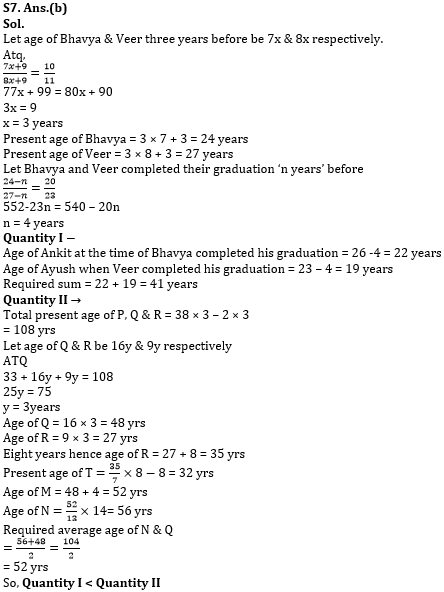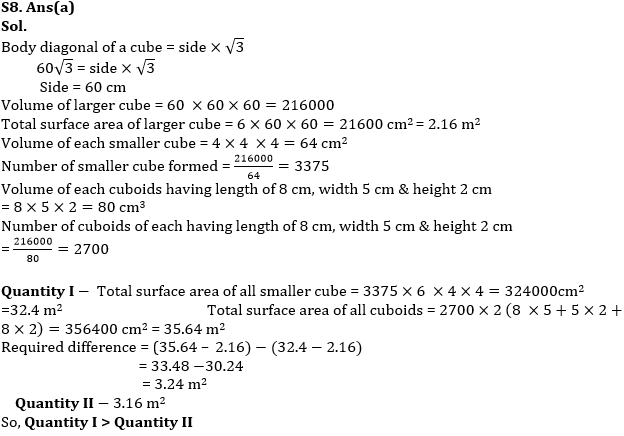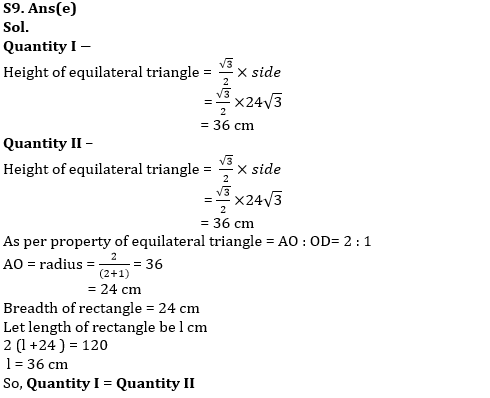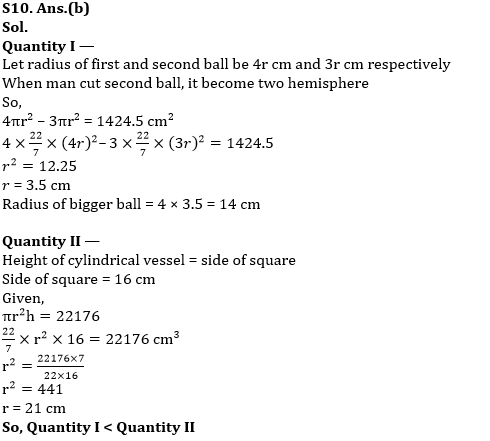#### Congratulations!Download Hindu Review of October 2021: Free PDF